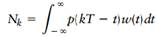Create an Account

Home / Questions / Determine the discrete time autocorrelation function of the noise sequence Nk defined by

Determine the discrete time autocorrelation function of the noise sequence Nk defined by

Determine the discrete-time autocorrelation function of the noise sequence {Nk} defined by Eq. (10.34)where w(t) is a white Gaussian noise process and the pulse p(t) corresponds to a root-raised cosine spectrum. How are the noise samples corresponding to adjacent bit intervals related?

Jul 30 2020 View more View LessSubscribe To Get Solution